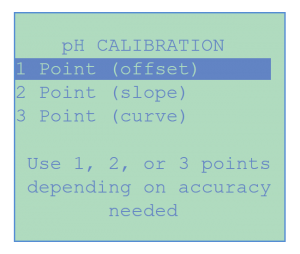# 10-Second Caibration

10-Second Calibration

CHEMTROL® sensors can be calibrated with 1, 2 or 3-Point calibration for origin, slope and curvature. Our user-friendly menu alloys the operator to conduct 1-point calibration within a few seconds. Calibration can be operated via PC, laptop or any mobile device. The easiest way is to use the CHEMTROL® App which available on both Android and Apple devices.
* It should be noted that there is no need to remove the probes off the by-pass assembly.Calibration Options

The CALIBRATION sub-menu is used to select the number of calibration points desired. Most applications require only 1- Point calibration but any number up to three can be selected. If more than 1-point calibration is selected, the operator needs to use calibrated buffer solutions. These sample solutions must be spaced sufficiently from one another to yield meaningful calibration values.

1. One-Point Calibration
When using 1-Point calibration, the conversion curve for the sensor readings is a straight line using the standard (default) slope built in the program. 1-Point calibration should be satisfactory for most applications.
The operator test the water with an appropriate test kit, the value obtained is then entered on the calibration screen as the new display value. The controller uses the calibration value that has been entered by the operator to calculate the origin “a” of the representative linear equation: DISPLAY = a + SLOPE * INPUT.
2. Two-Point Calibration
With 2-Point calibration, the operator needs to use two different solutions with values that are spaced widely enough to show significant differences in the slope of the calibration curve. The controller uses these values to compute the origin “a” and slope “b” in the equation: DISPLAY = a + b * INPUT.
3. Three-Point Calibration
With 3-Point calibration, the representative straight line is replaced by a second-degree polynomial curve. The operator needs three different solutions, again with values that are spaced widely enough to show differences in the curvature of the polynomial. The controller uses these values to compute the origin “a”, slope “b” and curvature “c” in the equation: DISPLAY = a + b * INPUT + c * INPUT*INPUT.

The CALIBRATION menu is common to all the functions that require sensor calibration, this includes Conductivity/TDS, pH, Temperature, ORP and PPM.

* Pressure and Flow have only direct 1- point calibration.